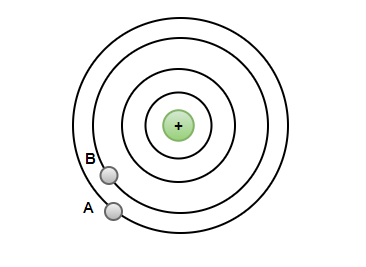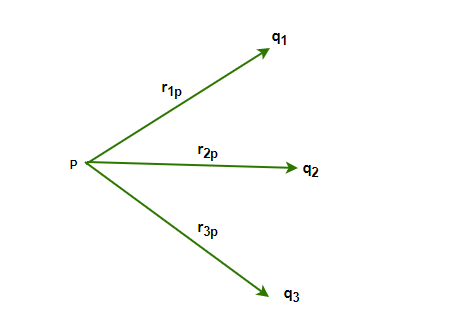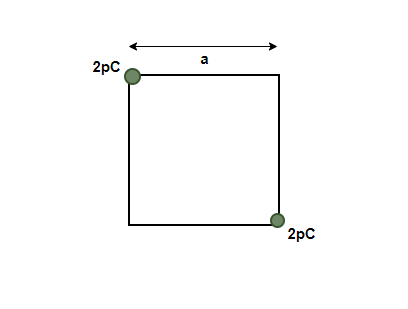GFG App
Open AppBrowser
Continue

# Electric Potential Due to System of Charges

The batteries in our phones and other devices sometimes have voltages written on them. Even in our homes, almost everything has a voltage rating that is written on it which tells us the safe operating range for these devices. But what is this rating? What is the physical intuition behind it? The voltage is usually an electric potential that charged bodies developing around them. This potential gives the idea of the energy stored in the charges that are in the vicinity of that charge. Usually, in real-life scenarios, there are many complex systems that deal with more than one charge. It is essential to study them and how to calculate the potential around the vicinity of such objects.

### Electric Potential

Electric potential is defined as the difference in the potential energy per unit charge between two places. To check the difference in the electric potential between two positions under the influence of an electric field, we ask ourselves how much the potential energy of a unit positive charge will change if that charge is moved from this position to the other position. It is denoted by V,

V = P.E/q

### Electric Potential Due to Point Charge

A point charge is given in the figure below. The concentric circles represent the equipotential. That means, that at all the points in a single surface, the potential is the same. The goal is to calculate the electric potential due to this point charge between two points A and B.Electric potential difference is also called voltage, and it is measured in the units of Volts.

voltageAB = electric potential differenceABThe potential has been defined as a difference; a formulation in terms of absolute potential is required. Let’s modify the above formulation to come up with this new definition. At infinite, the electric field and the potential are assumed to be zero. Now, the potential at every point will be calculated with respect to the infinite, and it will give an absolute value of the potential.

Now, rBand rA

voltageAB=Now, rB==### Potential Due to System of Charges

A single-point charge is rarely encountered in real life. Most of the systems found in real-life comprise multiple charges. For a system of point charges, the total potential at a point is given by the algebraic sum of the potential for individual charges at that point. The figure given below represents a system of point charges.For example, in a system containing charges q1, q2, q3 at a distance of r1P, r2P, and r3P from a point. Then, the potential at this point by individual charges will be given by,

V1V2,

V3The net potential due to these point charges is given by,In general,

For a system of point charges containing charges q1, q2, q3,q4 …. at a distance of r1P, r2P and r3P…. from a point.### Sample Problems

Question 1: Find the potential at a distance of 2 m from a point charge of 20pC.

The potential due to a point charge is given by,Here, q = 20 pC = 2 x 10-12C and r = 2 m.

Plugging the values into this equation,

V =⇒ V =⇒ V= 9 × 109 × 1 x 10-11

⇒ V= 9 x 10-2

Question 2: Find the potential at a distance of 5m from a point charge of 10pC.

The potential due to a point charge is given by,Here, q = 10 pC =10 x 10-12C and r = 5 m.

Plugging the values into this equation,

V =⇒ V =⇒ V= 9 × 109 × 2 × 10-12

⇒ V= 18 x 10-3

Question 3: Find the potential at a distance of 1 m due to a charge of 10pC and -2pC.

The potential due to a point charge is given by,Here, q1 = 10 pC = 10 x 10-12C, q2 = -2 pC = -2 x 10-12C and r = 1 m.

Since there are two charges in the system, the total potential will be given by the superposition equation.For two charges,Plugging the values into this equation,⇒ V= 36 × 10-3 V

Question 4: Find the potential at the center of a square of side a=2m due to a charge of 2pC and 2pC.The potential due to a point charge is given by,Here, q1 = 2 pC = 2 x 10-12C, q2 = 2 pC = -2 x 10-12

Since the square is of side a = 2. The length of the diagonal will be 2√2.

This distance of the point from both the charges will be √2.

Thus, r = √2

Since there are two charges in the system, the total potential will be given by the superposition equation.For two charges,Plugging the values into this equation,Question 5: Find the potential at the origin due to the charges 1pC and -1pC located at (0,1) and (2,0).

The potential due to a point charge is given by,Here, q1 = 1 pC = 1 x 10-12C, q2 = 2 pC = -1 x 10-12

The distance of these charges from the center is,

r1. = 1 and r2 = 2.

Since there are two charges in the system, the total potential will be given by the superposition equation.For two charges,Plugging the values into this equation,Question 6: Find the potential at the origin due to the charges 2pC and -10pC located at (0,3,4) and (0,0,5).

The potential due to a point charge is given by,Here, q1 = 1 pC = 1 x 10-12C, q2 = 2 pC = -1 x 10-12

The distance of these charges from the center is,

r1and r2 = 5.

Since there are two charges in the system, the total potential will be given by the superposition equation.For two charges,Plugging the values into this equation,My Personal Notes arrow_drop_up